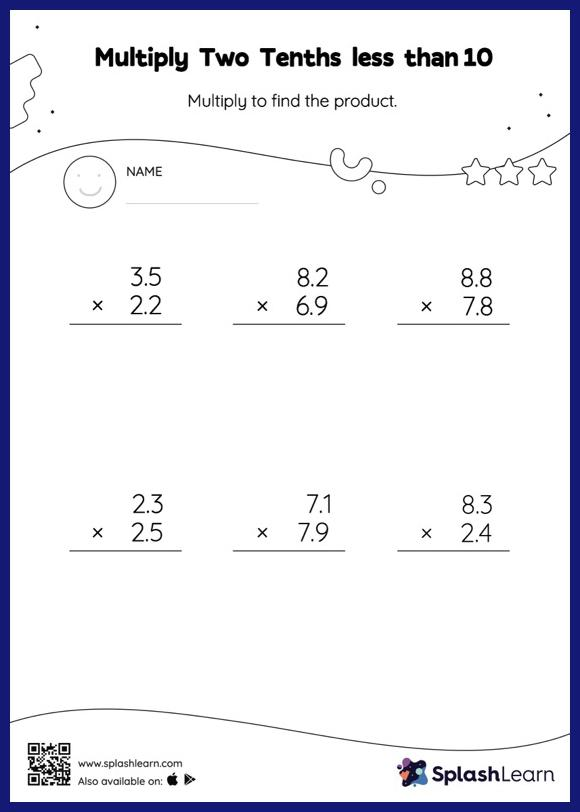# Multiply Two Tenths less than 10: Vertical Multiplication Worksheet

Home > Multiply Two Tenths less than 10: Vertical MultiplicationThe product of a tenth multiplied by another tenth will have two decimal digits. In the multiply two tenths less than 10 worksheet, students practice this idea by multiplying the numbers without considering the decimal points and then adding the decimal point two places from the right in the product. In each problem, the numbers are laid out in the vertical format. In this format, pair of digits in each successive place are tackled one by one. This helps students follow a structured approach.